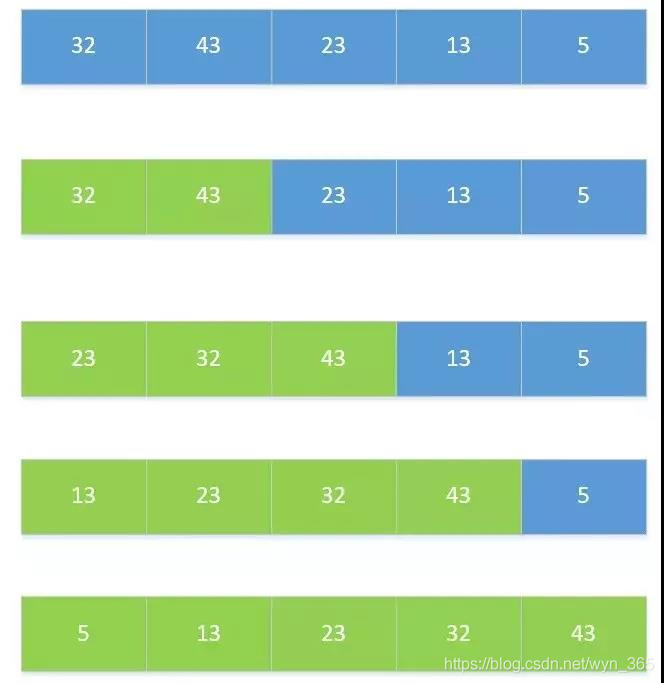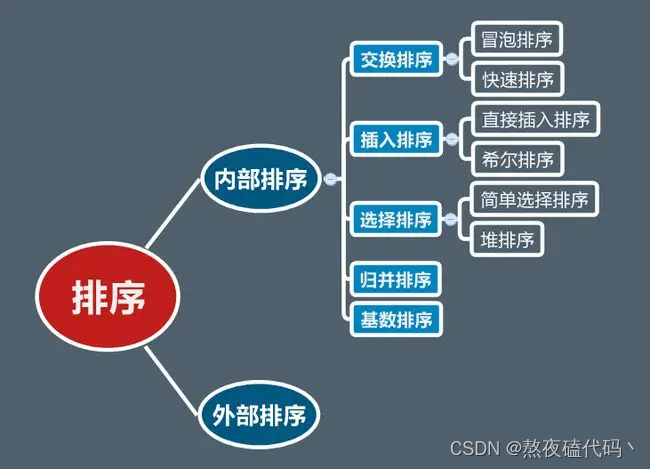# Java 实现的各种经典的排序算法小Demo

+关注继续查看

• 直接插入排序
• 折半插入排序
• 希尔排序
• 冒泡排序
• 快速排序
• 选择排序
• 桶排序
Demo下载地址

## 插入类算法

public static void DirectoryInsert(int []array,int length){
int p,i;
for(p=1;p<length;p++){
int temp=array[p];
i=p-1;
while(i>=0&&array[i]>temp){
array[i+1]=array[i];
i--;
}
array[i+1]=temp;
}
}

private static void Half(int[] array, int length) {
//p stands for the times of the sort
int left,right,mid,p;
for(p=1;p<length;p++){
int temp=array[p];
left=0;right=p-1;
while(left<=right){
mid=(left+right)/2;
if(array[mid]>temp){
right=mid-1;
}else{
left=mid+1;
}
}
for(int i=p-1;i>=left;i--){
array[i+1]=array[i];
}
array[left]=temp;
}
}

private static void Shell(int[] array, int length) {
// TODO Auto-generated method stub
int d=length/2;
while(d>=1){
for(int k=0;k<d;k++){
//to every sub,carry the direcly insert
for(int i=k+d;i<length;i+=d){
int temp=array[i];
int j=i-d;
while(j>=k&&array[j]>temp){
array[j+d]=array[j];
j-=d;
}
array[j+d]=temp;
}
}
d=d/2;
}
}

## 交换类排序算法

private static void Bubble(int[] array, int length) {
// TODO Auto-generated method stub
for(int i=0;i<length;i++){
//expeclally the end case is "length-i"
for(int j=1;j<length-i;j++){
if(array[j-1]>array[j]){
int temp=array[j];
array[j]=array[j-1];
array[j-1]=temp;
}
}
}
}

private static void BubbleBetter(int[] array, int length) {
// TODO Auto-generated method stub
boolean flag=false;
for(int i=0;i<length;i++){
//expeclally the end case is "length-i"
for(int j=1;j<length-i;j++){
flag=false;
if(array[j-1]>array[j]){
int temp=array[j];
array[j]=array[j-1];
array[j-1]=temp;
flag=true;
}
}
if(flag){
return;
}
}
}

private static void Fast(int[] array, int left, int right) {
// TODO Auto-generated method stub
if (left < right) {
int p = Partition1(array, left, right);
Fast(array, left, p - 1);
Fast(array, p + 1, right);
}
//      if (left < right) {
//          int p = Partition2(array, left, right);
//          Fast(array, left, p - 1);
//          Fast(array, p + 1, right);
//      }
}

private static int Partition1(int[] array, int left, int right) {
int pivot = array[left];
while (left < right) {
while (left < right && array[right] >= pivot) {
right--;
}
array[left] = array[right];
while (left < right && array[left] <= pivot) {
left++;
}
array[right] = array[left];
}
array[left] = pivot;
return left;
}

private static int Partition2(int[] array, int start, int end) {
int pivot = array[start];
int left = start, right = end;
while (left <= right) {
while (left <= right && array[left] <= pivot) {
left++;
}
while (left <= right && array[right] >= pivot) {
right--;
}
if (left < right) {
Swap(array[right], array[left]);
left++;
right--;
}
}
Swap(array[start], array[right]);
return right;

}

## 选择类排序算法

private static void Select(int[] array, int length) {
// TODO Auto-generated method stub
for(int i=1;i<length;i++){
int k=i-1;
for(int j=i;j<length;j++){
if(array[j]<array[k]){
k=j;
}
}
if(k!=i-1){
int temp=array[i-1];
array[i-1]=array[k];
array[k]=temp;
}
}
}

## 归并类排序算法

1）先把序列划分为长度基本相等的子序列
2）对每个子序列归并并排序
3）把排好序的子序列合并为最终的结果。

private static void MergeFunction(int[] array,int start, int end) {
// TODO Auto-generated method stub
if(start<end){
int mid=(start+end)/2;
MergeFunction(array, start, mid);
MergeFunction(array, mid+1, end);
Merge(array,start,mid,end);
}
}

private static void Merge(int[] array, int start, int mid, int end) {
// TODO Auto-generated method stub
int len1=mid-start+1,len2=end-mid;
int i,j,k;
//声明数组，分别保存子串信息
int[] left=new int[len1];
int[] right=new int[len2];
for(i=0;i<len1;i++){//执行数据复制
left[i]=array[i+start];
}
for(i=0;i<len2;i++){//执行数据复制
right[i]=array[i+mid+1];
}
i=0;j=0;
//执行合并操作
for(k=start;k<end;k++){
if(i==len1||j==len2){
break;
}
if(left[i]<right[j]){
array[k]=left[i++];
}else{
array[k]=right[j++];
}
}

//若array[start,mid]还有待归并数据，则放到array后面
while(i<len1){
array[k++]=left[i++];
}

//对array[mid+1,end]见得数据执行同样的操作
while(j<len2){
array[k++]=left[j++];
}
//释放内存操作
left=null;
right=null;

}JAVA排序算法
JAVA排序算法
21 0【排序算法】Java实现9大排序算法及其速度对比(附详细代码)
【排序算法】Java实现9大排序算法及其速度对比(附详细代码)
25 048 0【Java版】温习 8 种排序算法
【Java版】温习 8 种排序算法
33 096 0

Java高级编程7761162

JAVA开发手册1.5.0